Tutorial math and reading software for elementary and secondary arithmetic, basic math, algebra, geometry, precalculus plus GED, ABE, and CLEP preparation for elementary school, high school, college, adult education, and homeschool students.

# CLEP Test Preparation SoftwareCollege Mathematics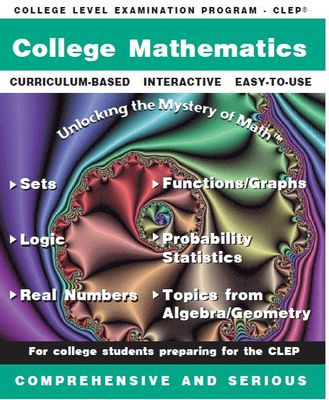Item# CLEPCM
Regular price: \$89.00
Limited Time Offer: \$44.50
Media type::

## Product Description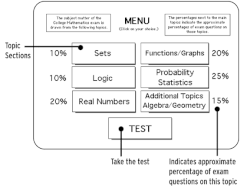This CLEP College Mathematics program provides you with a complete learning opportunity for success on the CLEP test College Math exam. The interactive clep math practice program is structured in the same order as the "Knowledge and Skills Required" on the CLEP College Mathematics examination.

Students preparing for college math placement exams will benefit from this program.

The "CLEP College Mathematics" exam contains the following topics: (1) Sets (10% of the exam)
Union and intersection;Subsets;Venn diagrams

(2) Logic (10% of the exam)
Truth Tables; Conjunctions, Disjunctions, Implications, Negations; Conditional Statements; Converse, Inverse, Contrapositive; Hypotheses, Conclusions, and Counterexamples

(3) Real Number System (20% of the exam)
Prime and composite numbers; Odd and even numbers; Factors and divisibility; Rational and irrational numbers; Absolute value and order

(4) Functions and Graphs (20% of the exam)
Properties and graphs of functions; Domain and Range; Composition of functions and inverse functions; Remainder theorem; Solving quadratic equations

(5) Probability and Statistics (25% of the exam)
Counting problems, permutations, combinations; Computation of probabilities of simple and compound events (compound probability); Simple conditional probability; Mean and median

(6) Additional CLEP test Topics from Algebra and Geometry (15% of the exam)
Complex numbers; Logarithms and exponents; Applications from algebra and geometry specifically on perimeter and area; Properties of triangles; The Pythagorean theorem; Parallel and perpendicular lines; Applications (Problem Solving)

This comprehensive program concludes with a practice CLEP exam (with the same content as the CLEP tests)which may be used as a tutorial with explanations or as a practice test for a grade -- weaknesses are analyzed so that the student can return to the body of the program for more practice.
Each of the main menu college math topics go to separate sub-menus of topics. Each of these topics contains a comprehensive lesson with interactive practice problems and complete solutions. All the topics required for success on the CLEP College Math exam are in this learning system. The practice CLEP College Mathematics test can be taken at the beginning of the study program to evaluate weak areas or after all the lessons in the program have been completed. There are a total of 34 lesson modules. CLEP exams provide students with limited time and funds to test out of required courses and gain college credit. The MathMedia CLEP college mathematics software provides college math learning opportunities with interactivity, math help, and a comprehensive practice CLEP exam.
FAQ
Typically, how long does it take to complete this particular program? Will this program prepare me for the college math clep test?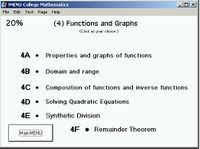Sub-menu of lessons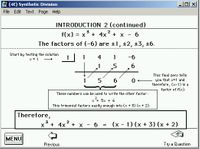Topics are introduced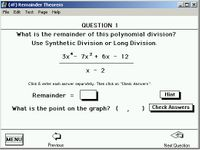Interactive Questions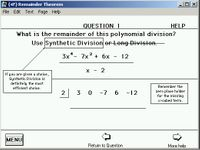Hints are a click away...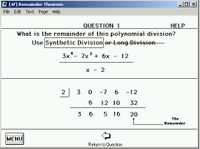The final solution is always available...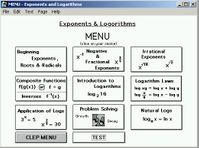Exponents & Logarithms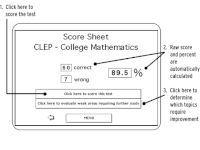The learning system concludes with a comprehensive practice CLEP TEST.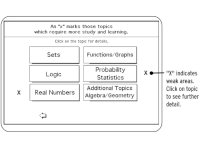Weaknesses are evaluated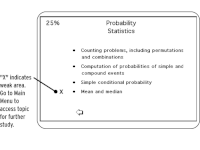Specific weaknesses are indicatedGet your degree!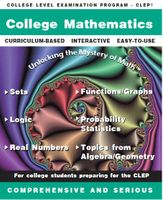Download CLEP College Mathematics Now! (\$89)Regular price: \$89.00Limited Time Offer: \$44.50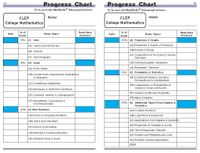Track your work on the Progress Chart (included)